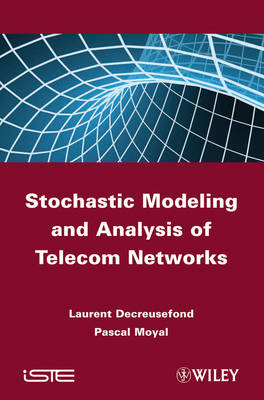•# Stochastic Modeling and Analysis of Telecom Networks (Hardback)

(author), (author)
£156.00
Hardback 398 Pages / Published: 09/03/2012
• We can order this

Usually dispatched within 1 week

This book addresses the stochastic modeling of telecommunication networks, introducing the main mathematical tools for that purpose, such as Markov processes, real and spatial point processes and stochastic recursions, and presenting a wide list of results on stability, performances and comparison of systems. The authors propose a comprehensive mathematical construction of the foundations of stochastic network theory: Markov chains, continuous time Markov chains are extensively studied using an original martingale-based approach. A complete presentation of stochastic recursions from an ergodic theoretical perspective is also provided, as well as spatial point processes. Using these basic tools, stability criteria, performance measures and comparison principles are obtained for a wide class of models, from the canonical M/M/1 and G/G/1 queues to more sophisticated systems, including the current hot topics of spatial radio networking, OFDMA and real-time networks. Contents 1. Introduction. Part 1: Discrete-time Modeling 2. Stochastic Recursive Sequences. 3. Markov Chains. 4. Stationary Queues. 5. The M/GI/1 Queue. Part 2: Continuous-time Modeling 6. Poisson Process. 7. Markov Process. 8. Systems with Delay. 9. Loss Systems. Part 3: Spatial Modeling 10. Spatial Point Processes.

Publisher: ISTE Ltd and John Wiley & Sons Inc
ISBN: 9781848212381
Number of pages: 398
Weight: 714 g
Dimensions: 239 x 158 x 27 mm